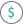#FV (PQL - xl)

This function returns the future value of an investment based on a present value, periodic payments, and a specified interest rate

• Library: PQL \ Spreadsheet \ Financial
• Compatibility: Any content (regardless of data source) in the Tabulate spreadsheet module
• Solve: This function can be used with the Solve plug-in: both as part of the objective function and constraint functions

#### Syntax

FV(rate, nper, pmt, OPTIONAL pv, OPTIONAL type)

##### Function Arguments
 Name Description Type Optional rate Interest rate expressed as percentage (per period) Number nper Total number of payment periods Number pmt Payment made each period Number pv Present value; if omitted, uses zero and the calculation is based on the paymt argument Number Y type Indicates when payments are due; at the end (0) or beginning (1) of the period; if omitted, the calculation uses the end (0) Number Y